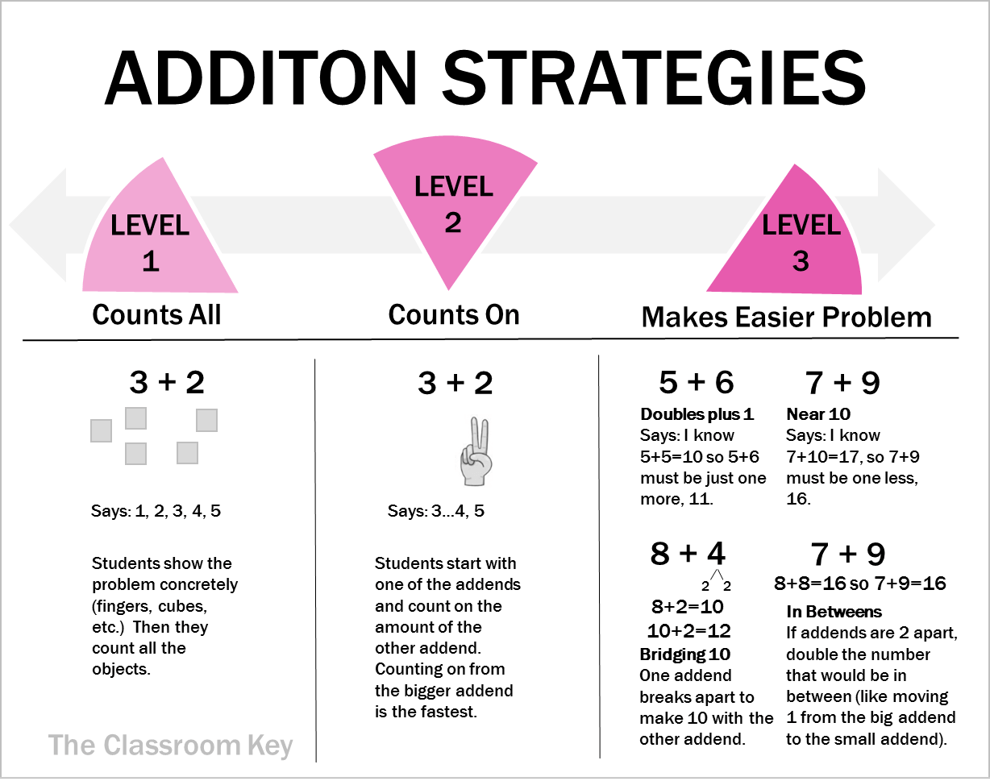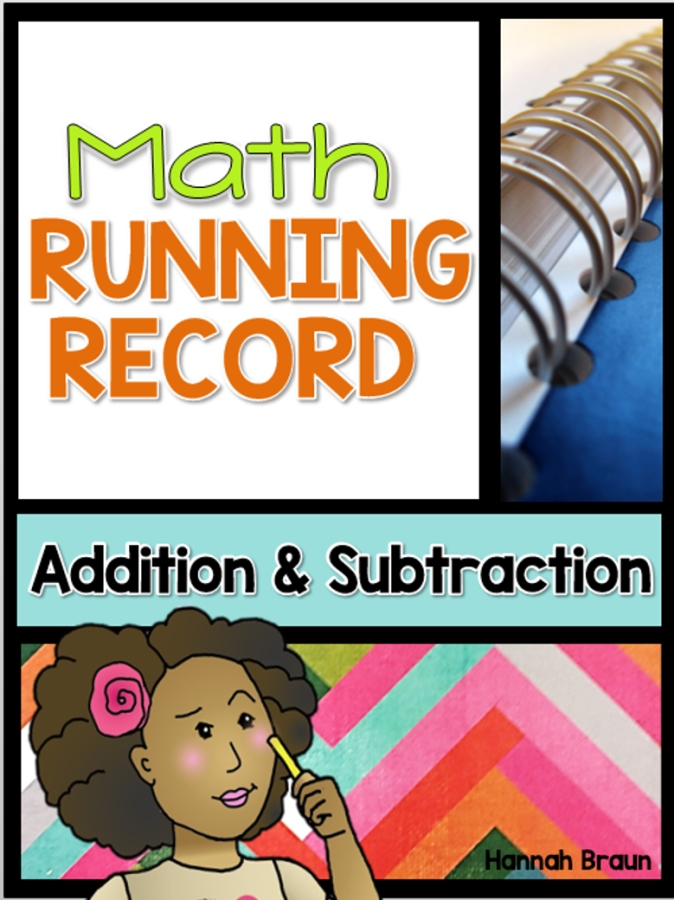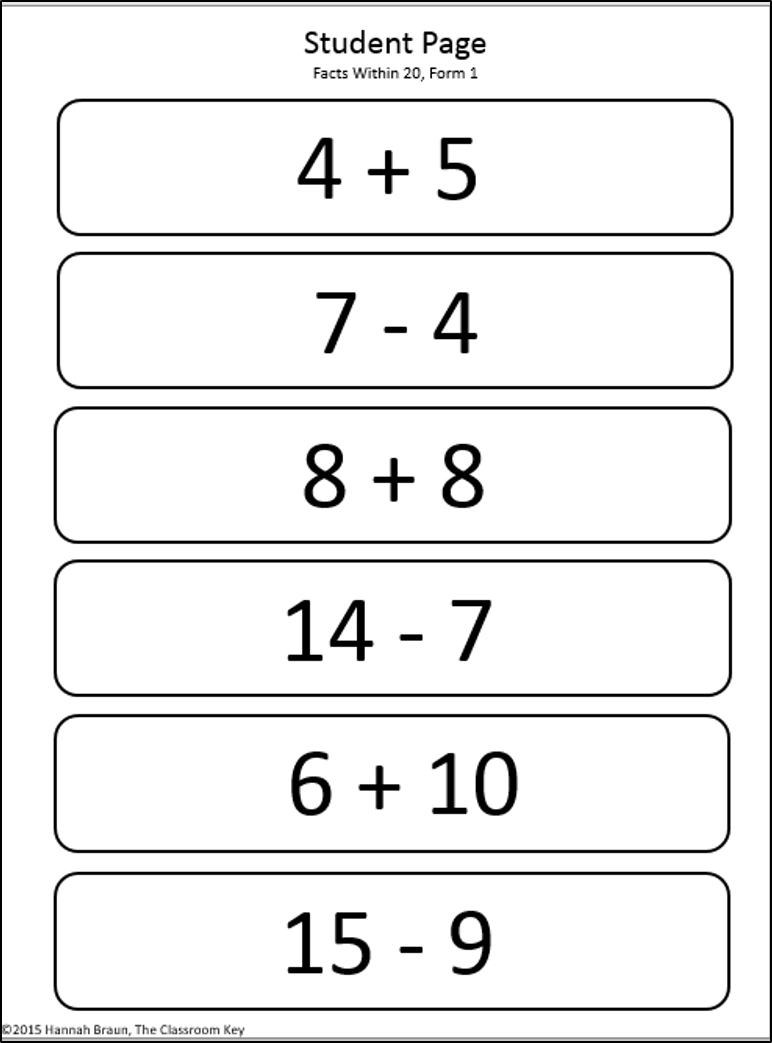Sharing is caring!

Ideas for teaching math fact fluency strategies in first and second grade.

## A Continuum of Math StrategiesMath strategies develop along a continuum (see the dashboard above).  We teachers can build students’ mathematical power by recognizing where they are on the strategy continuum, and helping them to move toward the most efficient strategies.The Institute for Mathematics and Education describes three levels of strategies for each operation (read more HERE).   When students are first learning an operation, they rely on concrete representations.  Eventually they learn faster and easier strategies.Level 1: Counts All – Students make a concrete representation of the problem by using some kind of manipulative or their fingers to show each addend.  Then they count all of the objects to find the total.  This is the least efficient strategy but a necessary starting place for…well, pretty much everyone!

Level 2: Counts On – Rather than counting every object, students just say one of the addends and then count on the amount of the other addend.  They will probably keep track of how many times they have counted on by using their fingers.  Once students are comfortable with this strategy they should be encouraged to always start by saying the biggest addend.  It’s a lot faster to count on the smaller addend.

Level 3: Makes an Easier Problem – There are lots of ways to make an easier problem.  It helps if students have already memorized doubles facts and ways to make 10.  Many of these strategies involve recomposing the addends in a way that’s easier to work with.  It may help students to model these strategies using 10 frames or cubes before they try to perform them mentally.  These strategies are typically the most efficient but students should be pushed into using them until they have a strong understanding of addition. (Have you heard of the “in-betweens strategy”? Read about it HERE)

Knowing HOW to use a math strategy is only the first step. Kids also need to know WHEN to use each strategy. I have a free addition strategies poster and strategy sorting activity for subscribers HERE## Subtraction StrategiesLevel 1: Takes Away- Just like with addition, the first strategy students will develop for subtracting involves modeling the minuend (the first number in the equation) using cubes or fingers and then physically taking away the subtrahend (the second number in the equation) to find the answer (the difference).  It gets tricky and slow when the minuend is higher than ten because students don’t have enough fingers anymore.  Before they get there, help guide them toward the next strategy…..

Level 2: Counts Up –  The next step is for students to start at the subtrahend and see how many “jumps” it takes to get up to the minuend.  They may keep track of these jumps on their fingers.  This is a more complex strategy than taking away because it requires that students understand they are calculating a difference rather than just taking away.  They begin to see that subtraction fits a part-part-whole model where the minuend is the whole and they are trying to figure out one of the parts (more on part-part-whole here).  In some situations counting up is the most efficient, such as 10 – 8.  Sometimes though, it’s more efficient to count back like when solving a problem like 10 – 2.  It’s important to spend time talking with your students about which strategy is the best when working on an equation.

Level 3: Makes an Easier Problem – There are lots of ways to manipulate numbers to make an easier problem.  Students are frequently more comfortable with addition facts.  If they understand that addition and subtraction are related, then they can use fact families (or related facts) to solve subtraction problems.  If they know that 3+3=6, then they also know that 6-3=3.  When student are comfortable with putting together and taking apart teen numbers, they can use the near 10 strategy.  When they approach a problem like 14-9 they can think, “I know that 14-10 would be 4.  That would be taking away 1 too many.  When I add that 1 back in, the answer is 5.”  Another way to make an easier subtraction problem is to bridge through 10.  This requires that students already know the combinations that make 10.  It works like this: If a student is solving 13-6, they break apart the subtrahend (6) so that they can take the minuend (13) down to ten first, and then use their memorized combinations of 10 to find the difference.  They would need to break the 6 into 3 and 3.  Then they can think 13-3=10, and 10-3=7.

To make an easier problem, students should know their doubles facts, combinations of 10, and how to use 10s and 1s to build and break apart teen numbers.  With these prerequisites, the “make an easier problem” strategies can be done mentally and are very efficient.

If you’re looking for a way to assess what math strategies your students are using (so you can guide them toward the next, most efficient ones!) check out my Math Running Record.  It’s a brief interview-style assessment that gives you both a quantitative score and qualitative information about your students’ addition and subtraction skills.  The data is useful for planning small groups, differentiated homework, goal setting at conferences, or even as an RTI assessment.#Sharing is caring!

Hannah BraunHannah Braun is a former teacher with 8 years of experience in the classroom and a master's degree in early childhood education. She designs engaging, organized classroom resources for 1st-3rd grade teachers.